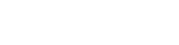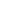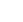Fairness
Overview
Fairness Types
Generation
View Seed Pairs
Verify Game Result

Provably Fair

We are laser-focused on providing our users with a fun and fair gambling experience. As such, we have developed state-of-the-art Provably Fair algorithms to prove that our games are fair.

The Provably Fair algorithm for each game is slightly different, but in essence, they all seek to provide you with confidence that the round was not tampered with as a result of you joining (in other words, we aren't rigging a game for or against any players). Each game's algorithm is described in detail below.

The Base of our algorythms is server seed, client seed and nonce. These are the values that guarantee our fairness. Client seed is string that you can define by youself. Server seed is SHA256 hash that we generate on server side. Nonce is a counter that starts from 0 and is incremented every time you bet. You can see and update you current seeds here.

Random numbers generation

The basic algorythm for our games is:
1. Get serverSeed, clientSeed and nonce.
2. Transform them into a float or floats in range [0,1).
3. Transform floats into game events by multiplying and then rounding them.

To transform serverSeed, clientSeed and nonce into floats we use function generateFloats, which inside uses function byteGenerator. Both functions can be found below. Count represents how many game events we need and cursor represents bytes offset.

```function generateFloats({
serverSeed,
clientSeed,
nonce,
cursor,
count,
}) {
const rng = byteGenerator({
serverSeed,
clientSeed,
nonce,
cursor,
});
const bytes = [];

while (bytes.length < count * 4) {
bytes.push(rng.next().value);
}

return _.chunk(bytes, 4).map(bytesChunk =>
bytesChunk.reduce((result, value, i) => {
const divider = 256 ** (i + 1);
const partialResult = value / divider;
return result + partialResult;
}, 0),
);
}```
```function* byteGenerator({ serverSeed, clientSeed, nonce, cursor }) {
let currentRound = Math.floor(cursor / 32);
let currentRoundCursor = cursor;
currentRoundCursor -= currentRound * 32;

while (true) {
const hmac = crypto.createHmac('sha256', serverSeed);
hmac.update(`\${clientSeed}:\${nonce}:\${currentRound}`);
const buffer = hmac.digest();

while (currentRoundCursor < 32) {
yield Number(buffer[currentRoundCursor]);
currentRoundCursor += 1;
}
currentRoundCursor = 0;
currentRound += 1;
}
}```

Shuffled numbers generation

Some games require non-repeating random numbers. To achieve this we use our implementation of Fisher-Yates algorythm.

```export const generateShuffledFloats = ({
serverSeed,
clientSeed,
nonce,
pick,
pickFrom,
}) => {
const floats = generateFloats({
serverSeed,
clientSeed,
nonce,
count: pick,
cursor: 0,
}).map((f, i) => Math.floor(f * (pickFrom - i)));

const allNumbers = new Array(pickFrom).fill(0).map((non, i) => i);
const res = [];

for (let i = 0; i < pick; i++) {
let float = floats[i];

let index = float;
for (let j = 0; j <= float; j++) {
if (allNumbers[j] === null) {
index++;
float++;
}
}
res.push(allNumbers[index]);
allNumbers[index] = null;
}

return res;
};```

Getting card from game event

To get card from game event we use eventToCard function.

```function eventToCard(event) {
const values = {
0: '2',
1: '3',
2: '4',
3: '5',
4: '6',
5: '7',
6: '8',
7: '9',
8: '10',
9: 'J',
10: 'Q',
11: 'K',
12: 'A',
};
const suites = {
0: 'D',
1: 'H',
2: 'S',
3: 'C',
};

const cardValue = (a / 4) | 0;
const cardSuit = a % 4;
return { lear: suites[cardSuit], value: values[cardValue] };
}```